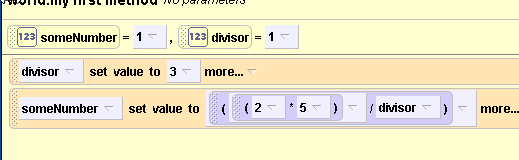# Math Expressions in Alice

Alice Supports many types of Math Operations.

For instance, let’s say that we have the variable someNumber  and it has a value of 2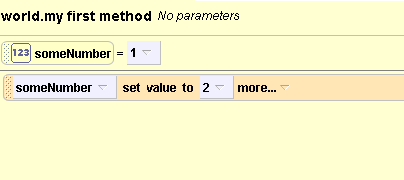## What if we want to multiply 2  by  0.5 ?

1. click on the down arrow next to “2” and select “math”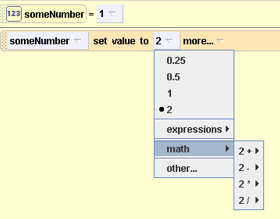2) select “2*”  which means “2 times ”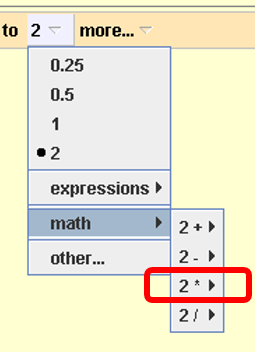3) Select “Other” for the second number “b”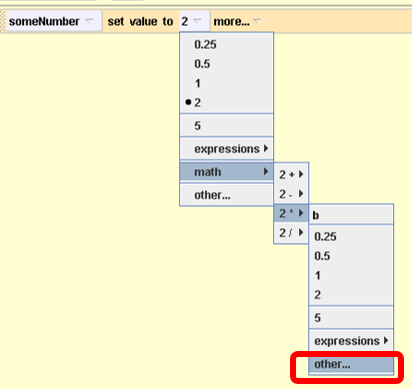4) The calculator appears so you can  choose 5

5)  Select “5”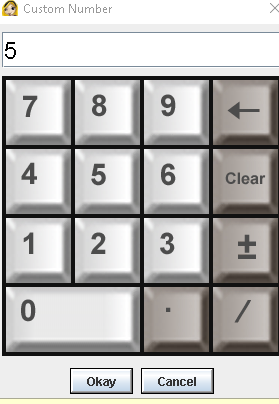6) Viola: You know have a math expression that represents 2 * 5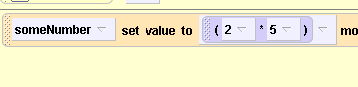8) Now let’s make a variable called “divisor” and then divide this expression by that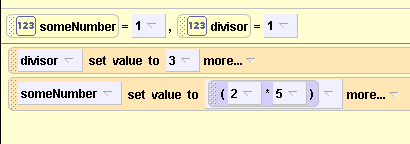9) Select the down arrow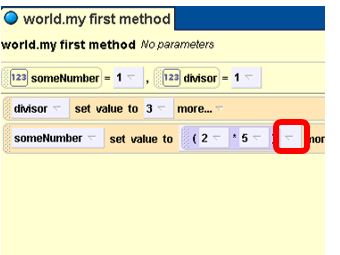10) select as shown: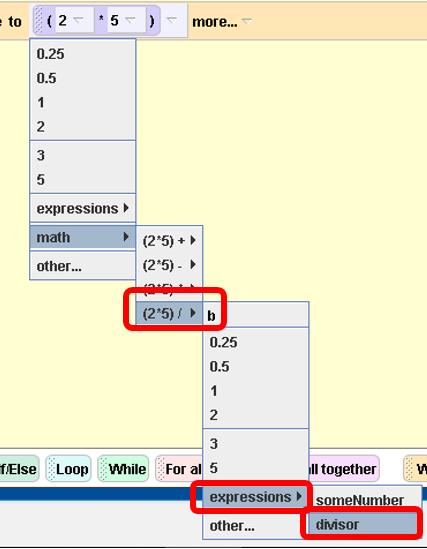11) Viola :